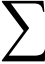# Sigma (Sum) Calculator

## What is Sigma?This symbol (called Sigma) means "sum up"

It is used like this:Sigma is fun to use, and can do many clever things. Learn more at Sigma Notation.

You might also like to read the more advanced topic Partial Sums.

## All Functions

### Operators

+ Addition operator Subtraction operator Multiplication operator Division operator Power/Exponent/Index operator Parentheses

### Functions

sqrt Square Root of a value or expression. sine of a value or expression cosine of a value or expression tangent of a value or expression inverse sine (arcsine) of a value or expression inverse cosine (arccos) of a value or expression inverse tangent (arctangent) of a value or expression Hyperbolic sine of a value or expression Hyperbolic cosine of a value or expression Hyperbolic tangent of a value or expression e (the Euler Constant) raised to the power of a value or expression The natural logarithm of a value or expression The base-10 logarithm of a value or expression Absolute value (distance from zero) of a value or expression the Factorial function!

### Constants

pi The constant π (3.141592654...) Euler's Number (2.71828...), the base for the natural logarithm